# Gem Island

Are you fresh off the back of the long weekend and looking to sink your teeth into some combinatorics and induction? Great then you’ve come to the right place so pull your discrete mathematician stocking over your head and let’s get started.

Welcome to Gem Island! Home to `N` happy islanders. Each of which has exactly one gem. Each night something peculiar happens. One of the gems on the island is chosen uniformly at random and it splits creating a new gem for the owner. This process repeats each night for `D` nights. Here is an example of all 6 situations that could arise if there were (`N=2`) islanders after (`D=2`) nights. Here we’ve coloured the first islander’s gems red, the second green and the vertical bar separates the islanders to make things easier to read.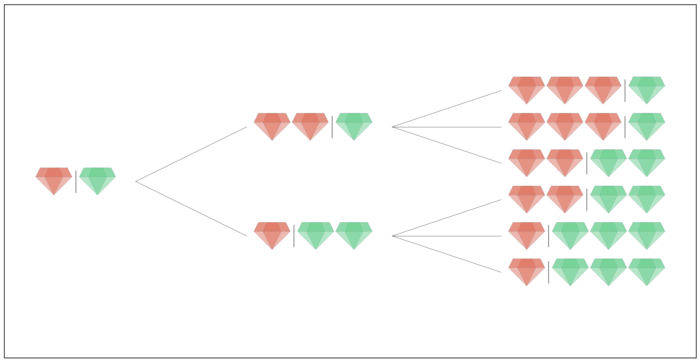We are interested in the expected value, of the number of gems, the `R` richest islanders have. In the case above the `R=1` richest islanders has on average `(3 + 3 + 2 + 2 + 3 + 3) / 6` gems. Where as the `R=2` richest islanders would have`( (3+1) + (3+1) + (2+2) + (2+2) + (1+3) + (1+3) ) / 6 = N + D`. In fact if `N <= R` the answer is going to be `N + D` because at the end of the process there is always the same number of total gems on the island. So we have our problem and it’s completely described by just three numbers `N`, `D` and `R`.

Before looking for a solution to the actual problem let’s simulate out a few more examples. Here’s what things would look like with `N=3` islanders after `D=2` nights.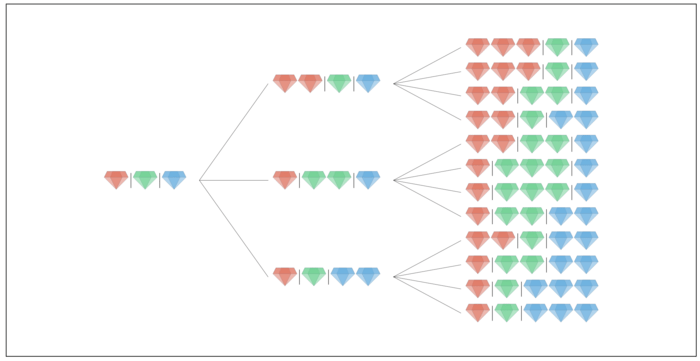Here’s the situation for `N=3` and `D=3`. Things are exploding a bit and there are 60 final distributions of gems in the leaves of the tree.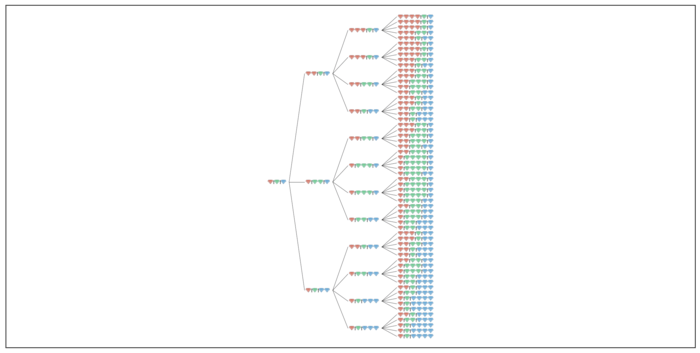What about `N=4` islanders after `D=3` days? Just showing the final distributions, there are 120: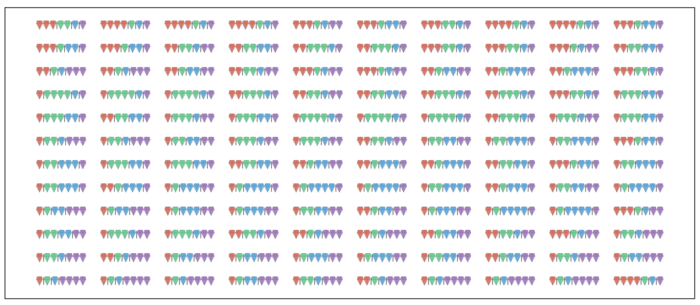You get the idea. So before attempting to solve this problem it’s interesting to consider 3 related questions.

• How many distributions of gems are possible?
• How many of them are unique?
• How many of them are unique permutations (not permutations of others)?

Using the example of `N=2` and `D=2`:There are 6 total distributions:
`(3,1)`, `(3,1)`, `(2,2)`, `(2,2)`, `(1,3)`, `(1,3)` of which 3 are unique: `(1,3)`, `(3,1)`, `(2,2)` and 2 are unique permutations: `(3,1)` and `(2,2)` because `(3,1)` is a permutation of `(1,3)`. Now we could stop there and it would be fun to come up with answers to those three questions.

Let’s answer that first questions because the diagram above gives a good illustration. How many distributions of gems are there are there for a given `N` and `D`? Well there are `N` possible transitions from the first state, then `N+1`, then `N+2` and so on, `D` times. So the number of final distributions of gems that are possible are `N * (N+1) * ... * (N+D-1)`. It looks like numbers of this form are called rising factorials: `RF(N, D) = N * (N+1) * ... * (N+D-1)`.

## Solution 1 - O(N!) #

This actually gives us our first solution to the problem. If we could count the number of gems the `R` riches islanders have in each of the `RF(N, D`) distributions we could divide by that number and that would give us an answer. Coding this up requires completely enumerating all the final distributions and then summing up the `R` highest value in each one. Slow and steady can win the race:

``````def rf(N, D):
"""
rising factorial - returns n * (n+1) ... * (n+d-1)
"""
ret = 1
for x in range(D):
ret *= (x + D)
return ret

def recurse(N, D, R, gems):

if D == 0:
counts =  * N
for g in gems:
counts[g-1] += 1

# return the sum of gems R richest islanders have
return sum(sorted(counts)[::-1][:R])

ret = 0
for g in gems:
gems.append(g)
ret += recurse(N, D - 1, R, gems)
gems.pop(-1)
return ret

if __name__ == '__main__':
N, D, R = 2, 2, 1
# gems stores which islanders owns which gems.
# g[a] = b mean the a'th gem is owned by islander b
# gems = [1, 2, ..., n]
gems = [ x for x in range(1, N + 1)]
sumr = complete_enumeration(D, N, R, gems)
expected = sumr / rf(N, D)
print(expected)
``````

While correct this solution is going to be offensively slow, in the order of `O(N!)` so let’s try and find something faster. Let’s look back at all the distributions possible from `N=3` and `D=3` again: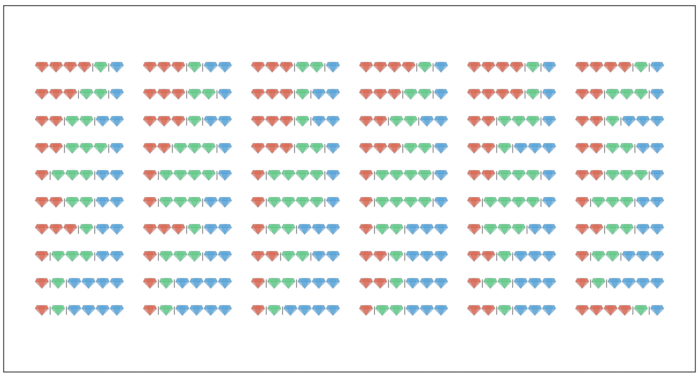Notice that there are 60 different distributions but lots of them are duplicates and there are only 10 unique distributions: `(4,1,1)`, `(1,4,1)`, `(1,1,4)`, `(2,2,2)`, `(1,2,3)`, `(1,3,2)`, `(2,1,3)`, `(2,3,1)`, `(3,1,2)` and `(3,2,1)`. How do we compute that `10` directly from `N` and `D`? Firstly the sum of elements is always `D+N`. Also, and surprisingly ,. each of these unique distributions is also equally likely and in the case of `N=D=3` will appear 6 times. This is not super obvious so we should probably try and prove that as it would mean we only need to consider many fewer distributions.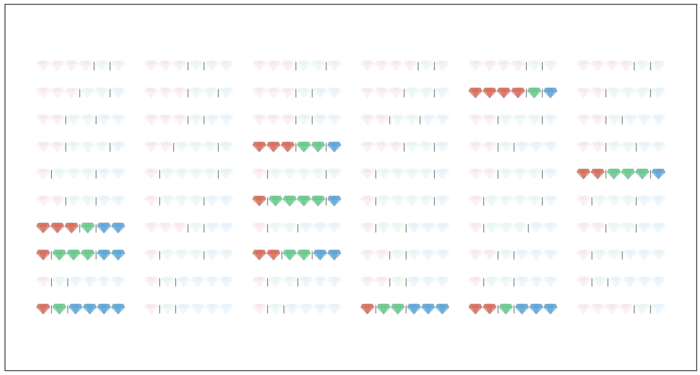## Aside: Proof by Induction #

Proving that each of the unique distributions is equally likely can be done by induction on `D`. For `D=0` there is only the initial distribution where each islander has one gem `(1, 1, ... , 1)`. For a higher value of `D` there are `N` potential ways to get to that distribution which we can call `(g1, g2, ... ,gN)`. These `N` ways are each from a distribution with one less gem. The probability that we get from a previous distribution, namely `(g1, g2, ..., gK-1, ..., gN)` to `(g1, g2, ... ,gN)` is `(gK - 1) / (N + D - 1)` as one of the `gK - 1` has to be the one that splits. Since the probability for each distribution for `D-1` is some fixed `P` by the induction hypothesis then the total probability is `(P * D) / (N + D - 1)`.

## Solution 2 - O(N4)#

So how many unique distributions are there? Fortunately there is a closed form for this using combinatorics. Think of creating a distribution by having a bag full of labels with each islanders name on it. There are many labels with each islanders name on it too. Then take `D` labels from the bag and each time you do that corresponds to that islander getting a gem. The key here is repetition is allowed. The number of ways of choosing `D` items from a set of `N` is `N choose D = choose(N, D)`. While allowing repetition is done using the multiset coefficient `multichoose(N, D) = choose(N + D - 1, N)`. So in the above example with `N=3` and `D=3`: `multichoose(3, 3) = choose(3+3-1, 3) = 10`. Now since all these unique distributions are equally probably we can calculate the sum of the gems the `R` richest islanders have in each and divide by `choose(N+D-1, D)`. The values of `choose(N, K)` can be precomputed using the recurrence relation `choose(N, K) = choose(N-1, K) + choose(N-1, K-1)`:

``````# Precompute the values of n choose k
MAX = 50
nCk = np.zeros((MAX, MAX), dtype='uint')
nCk[:, 0] = 1

for n in range(1, MAX):
for k in range(1, n + 1):
nCk[n][k] = nCk[n-1][k] + nCk[n-1][k-1]

def choose(nCk, n, k):
return nCk[n][k]

def multichoose(nCk, n, k):
return choose(nCk, n + k - 1, k)
``````

Cool so we’ve answered the second of our related questions above. Now let’s try another solution. Instead of enumerating all the distributions - knowing that each of the unique ones is equally likely lets count the number of ways of allocating `D` gems to the islanders and also the total number of gems the `R` richest islanders have. Imagine trying to do this in a structured way that you can easily describe what has already being allocated without having to store all the previously allocated gems as we did in the previous solution with the `gems` list. The reason to do this is if we can describe the state using fewer variables and additionally relate the state to other states we can typically compute the value efficiently using dynamic programming.

In our previous solution our function took parameters `N, D, R` and also a `gems` list which could have contained `RF(N, D)` (many) different values. That list is a problem. Imagine instead that we are counting the number of unique distributions of gems the islands could end up with. Let imagine we are allocating gems in descending order so if we are allocating 3 gems to some set of islanders we don’t need to worry about whether any islanders after that though would have more than 3 gems. We will call the number of gems we are currently allocating `i`. Let’s also remember how many gems we have left to allocating knowing that we need to allocate `N+D` gems in total. Let call that `j`. And finally let’s remember the number of islanders who still need to have gems allocated to them knowing that each islander needs at least one gem, at more `D+1` gems and the total gems should equal `N+D`. Let’s call that `k`. So now we have some partial state describing the number of ways of allocating `i` or fewer gems to `k` islanders having `j` gems left to allocate as `F[i][j][k]` and we are seeking `F[D+1][D+N][N]`. This may not seem helpful at the moment but the important this to remember is we are describing something similar to the `recurse` function in the previous solution with a drastically reduce number of states. ``(D+1)*(D+N)*N` is far smaller than `N*D*RF(N, D)`.

But we need a way to compute `F[D+1][D+N][N]` without visiting states more than once The key to doing this is describing `F[i][j][k]` in terms of other states. If we are at `F[i][j][k]` then there is some subset (of size say `s`) of the `k` remaining islanders that are each going to get `i` gems. How many subsets are there well there are `choose(k, s)`. Then we are done allocating `i` gems and transition to state `F[i-1][j - i*s][k - s]`. So our recurrence is: `F[i][j][k] = choose(k, s) * F[i-1][j - i*s][k-s]` summed over all valid `s`. We can keep another state `G[i][j][k]` to collect the sum (instead of the count) of the gems the `R` richest islanders have and compute it in a very similar way. This all leads to a nice compact solution which run ins `O(N^4)`, much faster than our previous one but still very slow. Also while it’s a bit mind-bending to try and look at the code directly and understand what is going one knowing what `F[i][j][k]` and `G[i][j][k]` store and their recurrence relation is all that is needed. The rest is just bounds checking.

``````def dynamic_programming(N, D, R):

F = np.zeros((D+1+1, D+N+1, N+1), dtype='double')
G = np.zeros((D+1+1, D+N+1, N+1), dtype='double')

F = 1

for i in range(1, D + 1 + 1):
for j in range(0, D+N+1):
for k in range(0, N+1):
for s in range(0, min(k + 1, 1 + j // i) ):
kCs = choose(k, s)
F[i][j][k] += kCs * F[i-1][j-s*i][k-s]

# compute the contribution to the sum of gems
# R richest islanders have as gems are allocated
# in descending order
if R - (N-k) > 0:
factor = (i-1) * min(R-(N-k), s)
else:
factor = 0

G[i][j][k] += kCs * G[i-1][j-i*s][k-s]
G[i][j][k] += kCs * factor*F[i-1][j-s*i][k-s]

f = F[D+1][D+N][N]
g = G[D+1][D+N][N]
return g / f + R

if __name__ == '__main__':
N, D, R = 10, 10, 2
expected = dynamic_programming(N, D, R)
print(expected)

``````

Note that the final expected value is always at least `R` because each islander starts with one gems. So we can add that contribution at the end and assume islanders start with zeros gems each where as in fact they start with 1.

## Solution 3 - O(N3)#

We’re making good progress here. We’ve answered two of our related questions and gotten a solution that will run in `O(N^4)`. So let’s look at the third related question, how many unique distributions are there (excluding permutations). We can compute this using multinomial co-efficients. This is like asking how many unique permutations are there of the word `MISSISSIPPI`. There are `11!` permutations but many are repeated. So we divide by the number of ways of permuting the repeated groups `I`, `S` and `P` which is `4!`, `4!` and `2!`. So `11! / (4!*4!*2!) = 34650` is the number of unique permutations of the letters `MISSISSIPPI`. We can do the same thing with gems distributions let again look back at the small example with `N=D=2`:There are `6` distributions and only two unique permutations: `(3,1)` and `(2,2)`. There is `1 = 2! / 2!` unique permutations of `(2, 2)` and there are `2 = 2! / (1!*1!)` unique permutation of `(3, 1)` for a total of `3` unique distributions. This is sort of helpful at this point but what we really need to do is look at how to describe our state with fewer or smaller variables. We previously allocated amounts of gems from largest to smallest. Let’s look at things a different way and start with all islanders having exactly 1 gem. There is some subset of islands that will receive no more gems during the process they will end the process with 1 gem. But there is a subset which will gain 1 or more gems. So let’s again describe our state of the number of ways of allocating `i` islanders with `j` gems as `F[i][j]` and then our recurrence relation becomes `F[i][j] = choose(i, k) * F[k][j-k]`. This is much clearer and describes the same information. Coding this up gives a solution that is an order of magnitude faster at `O(N^3)`

``````def dynamic_programming(N, D, R):

F = np.zeros((N+1, D+1), dtype='float')
G = np.zeros((N+1, D+1), dtype='float')

F = 1.0

for i in range(N + 1):
for j in range(D + 1):
for k in range( min(i, j) + 1):
iCk = choose[i][k]
F[i][j] += F[k][j-k] * iCk
G[i][j] += (G[k][j-k] + min(r, k) * F[k][j-k]) * iCk

return F[N][D] / G[N][D] + r
``````

Now we’ve answered our three related questions and can confidently count the number of distributions, unique distributions and unique permutations. We’ve also got three different and increasingly faster solutions to the problem. With our understanding let’s try and make one final attempt.

## The Illusive O(N2) Solution #

At this point you’ve probably drawn lots of those tree diagrams and counted things many times so let’s look at the problem in a different way. Let `S(N, D, R)` be the sum of the number of gems the `R` richest islanders have at the end of the process. So for `N=D=2` and `R=1`, `S(N, D, R)` equals `16` and we can divide by number of unique distributions `multichoose(N, D) = 6` which gives the answer `2.666 ...`. We know how to compute the `multichoose` so how do we compute `S(N, D, R)?`

Here’s an analogy. Imagine if I said compute the expected value of sequence `1, 4, 2`. You would add them up and divide by `3`. That would work. Now instead of giving you the actual values `1, 4, 2` I gave you a function `V(K)` and told you that `V(K)` was the number of values in the sequence that are greater than or equal to `K`. So `V(0) = 3`, `V(1) = 3`, `V(2) = 2`, `V(3) = 1`, `V(4) = 1` and `V(5) = 0`. How would you compute the expected value? Well you could just sum up the values of `V(K)` for all `K` from `1` upwards which would equal `3 + 2 + 1 + 1 = 7` and again divide by `3`. What’s nice about this approach is if I asked you to sum the `R=2` largest number in that sequence you could just do that by summing `min(R, V(K))` instead of `V(K)` and get `2 + 2 + 1 + 1 = 6` which is the correct answer. You don’t need to sort any thing or know anything about the ordering.

Knowing that let’s define `S(N, D, R)` as the sum of all `min(K, R)*V(K, Y)` and this `V(K, Y)` is the number of unique distributions of gems where exactly `K` have at least `Y` gems each. If we also say that `W(K, Y)` is the number of unique distributions of gems where at least `K` islanders have at least `Y` gems each. Note the difference between `V` and `W`. Then `V(K, Y) = W(K,Y) - W(K+1),Y)` So we just need to find a way to compute `W(K, Y)` which can be done almost directly. First we choose the `K` islanders that will each be getting `Y` gems and then allocate the remaining gems as we please. `K` islanders can be chosen from `N` as `choose(N,K)` and then the remaining `N + D - (Y-1)*K` gems can be allocated to any of the islands in `choose(N + D - (Y-1)*K - 1, N + D - (Y-1)*K)` ways. The only problems is that this will lead to some double counting so we need to use the inclusion-exclusion principle to compute `W(K, Y):`

``````def W(N, D, K, Y):

ret = 0
for L in range(K, N + 1):
if D - L*(Y-1) >= 0:
incl_excl =(-1)**(L-K)*choose(L-1,K-1)
x = incl_excl*choose(N,L)*choose(N+D-L*(Y-1)-1,D-L*(Y-1))
ret += x
return ret
``````

And having `W` we can compute `V`:

``````def V(N, D, K, Y):
return W(N, D, K, Y) - W(N, D, K+1, Y)

``````

and then we can sum up the `V` table to compute `S` and hence our expected value:

``````def S(N, D, R):

ret = 0
for K in range(1, N + 1):
for Y in range(1, D + 2):
ret += min(R, K) * V(N, D, K, Y)

return ret

expected = S(N, D, R) / choose(N + D - 1, D)
``````

This solution will run in `O(N^2)` and compute the correct answer. I really like problems like this. They are entirely described by some very small inputs, in this case just three numbers `N`, `D` and `R` and admit a simple yet prohibitively slow solution by directly simulating the process. But digging in a bit more with some combinatorics yields increasing elegant and faster solutions. Is there a faster solution than `O(N^2)`? I don’t know, maybe there is even a closed for solution to this problem which I would love to see if it exists!

This post is based on a question from ICPC World Finals 2018.